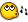# 36-base number system

## Recommended Posts

When I input for example the number 38 to script, it would give me code B1. When given the number 0, output would be 1. Give the number 11 and output would be A. So the number system would be based on 36 symbols (ABCDEFGHIJKLMNOPQRSTUVWXY1234567890). Got it? I have idea of defining all of the numbers up to 36 and then reseting some variable and adding +1 to the next variable and... Then I remembered that there's some table things, where you can fill the table with any given symbols and access to them easily afterwards. But I have no idea what would be the next step. So how would I manage to do working 36-based number system?

Edited by ValdeZ
##### Share on other sites

When I input for example the number 38 to script, it would give me code B1. When given the number 0, output would be 1. Give the number 11 and output would be A. So the number system would be based on 36 symbols (ABCDEFGHIJKLMNOPQRSTUVWXY1234567890). Got it?

No, I don't get it since your table does not correspond to the output you want.

But put your table in an array. Use mod to figure out number positions and do a lookup in the array.

##### Share on other sites

No, I don't get it since your table does not correspond to the output you want.

But put your table in an array. Use mod to figure out number positions and do a lookup in the array.

I messed up a bit. Meant:

input 1, output 1

input 11, output B

input 35, output 10

input 36, output 11

I have put A-Z and 0-9 symbols to one array. But how I supposed to do hmm, maths with these numbers? For example 35+1 (this is 10 number based) and output should be 11? I thought that I could make something like

```\$variable10based

If \$variable10based = 34

\$variable10based2 = \$variable10based2 + 1

\$variable10based = 0

; and then... no idea because this doesn't look good```I can't

##### Share on other sites

This is exactly reverse of what you want.. Just to make it more interesting for you.```MsgBox(0, "", _36BaseToDec("AHX") )

Func _36BaseToDec(\$s_Number)
Local \$s_Return = 0
\$s_Number = StringUpper(\$s_Number)
\$s_Split = StringSplit(\$s_Number,"")
If \$s_Split <= 0 Then
SetError(1)
Return 0
Else
For \$s_X = \$s_Split To 1 Step -1
\$s_Base = Asc(\$s_Split[\$s_X])
Switch \$s_Base
Case 0x30 To 0x39
Case 0x41 To 0x5A
Case Else
SetError(2)
Return 0
EndSwitch
;           MsgBox(0, "", "Step: " & 10^((\$s_X-\$s_Split)*-1) & @CRLF & _
;           "Char: " & \$s_Split[\$s_X] & @CRLF & _
;           "This chars value: " & \$s_Add / (10^((\$s_X-\$s_Split)*-1)) & " * " & 10^((\$s_X-\$s_Split)*-1) & " = " & \$s_Add  & @CRLF & _
;           "new return value: " & \$s_Return)
Next
SetError(0)
Return \$s_Return
EndIf
EndFunc```
##### Share on other sitesThanks! This may take some time to learn what those commands do, but then I believe that I can do what I wantEdited by ValdeZ
##### Share on other sitesThanks! This may take some time to learn what those commands do, but then I believe that I can do what I wantNo problem, ValdeZ. The mathematics behind these are not hard to understand, only I haven't been able to find a website that explains them properly.. Do you know the results from this function are correct?

##### Share on other sites

That link seems to be wonderful! Gee, thanks! :">

## Create an account

Register a new account

• ### Recently Browsing   0 members

×

• Wiki

• Back

• #### Beta

• Git
• FAQ
×
• Create New...